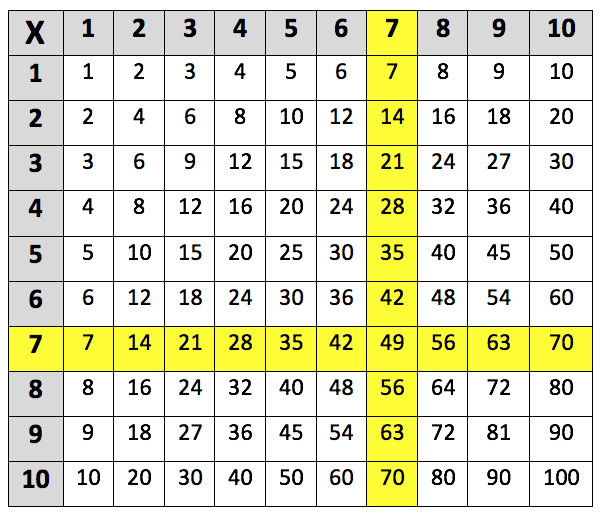Learning the 7 times table. The 7 times table up to 7 x 10 is highlighted in the multiplication grid below:This is the 7 times table up to 7 x 12:
7 x 1 = 7
7 x 2 = 14
7 x 3 = 21
7 x 4 = 28
7 x 5 = 35
7 x 6 = 42
7 x 7 = 49
7 x 8 = 56
7 x 9 = 63
7 x 10 = 70
7 x 11 = 77
7 x 12 = 84

## When do children learn the 7 times table?

During Year 4, children will learn the 6, 7, 9, 11 and 12 times tables. By the end of Year 4, children should have been taught all the times tables up to the 12 times table. Teachers will start giving children harder multiplications to complete, these will include multiplying three-digit numbers by a one-digit number (such as 150 x 3 = 450).

Children will utilise their knowledge of times tables in Years 5 and 6 when learning to do other mathematical operations.

## How are children taught the 7 times table?

There are many different methods of learning the times tables to explore, some teachers try and make it fun by getting children to visualise how a number is multiplied.

Teachers may get children to use Unifix cubes to make number sticks which can help them solve mathematical problems. For example, teachers could ask children to make a number stick which represents the answer to 7 x 2, by joining together two number sticks of 7 cubes each and then counting how many cubes there are in total (14). The fact that children can see and hold these number sticks can make learning times tables easier, as it means children can visualise the process of multiplication.

## How to help children with the 7 times table?

Sometimes asking children to fill out an empty multiplication grid is a useful way of checking which times tables or specific multiplications that they are finding difficult.

For example, it is quite common for children to struggle with the 7 times table. This is because 7 is a prime number and so the final digit 7 only appears again in 7 x 10 (=70).

Also, the 7 times table does not have any helpful patterns like the times tables for even numbers do, nor are there any memorable rules like with the 3 and 9 times tables.

There is an easy way of helping children remember that 7 x 8 = 56, as they can learn the sequence: 5, 6, 7, 8. The answer is the two numbers that come before the numbers being multiplied.

It can also be useful to remind children that they can reverse the order of a multiplication if this makes it easier to calculate. For example:

7 x 5 is the same as 5 x 7 (both = 35)

This is helpful as children can use the times tables that they are more confident with (such as the 5 times table), whilst also practicing the ones they find more difficult.

It is important to remember that learning the 7 times table is likely to take children longer than the other times tables. Therefore, it is a good idea to keep trying different methods until one seems to work for your child.

## How does Learning Street help children with the 7 times table?

Repetition is key to learning your 7 times table, and in the Learning Street 8 year old courses, there is constant focus on always practicing times tables as this is the best way to improve. This is through times tables tests (to improve accuracy), tables races (to improve time), mental mathematics, maths problem solving and other fun activities such as mystery pictures.

Without a good knowledge of times tables, it can lead to problems in SATs, GCSEs and A Levels.

## Our Courses

Click through to review the courses we have available

##### Get Started

Learning Street structured courses make home study easier and more successful. There's no need to wonder which books to buy or what to do next. Everything is planned for you.

• Proven to deliver excellent results
• Used by families, private tutors and schools
• Fully planned and structured